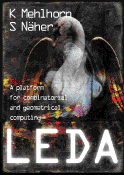The LEDA Platform of Combinatorial and Geometric Computing## The LEDA Platform of Combinatorial and Geometric Computing

### K. Mehlhorn and St. Näher

Cambridge University Press, 1999.

#### Corrections and Remarks

• Chapter 1, Introduction, ps, pdf
• Chapter 2, Foundations, ps, pdf
• Chapter 3, Basic Data Types, ps, pdf
• Chapter 4, Numbers and Matrices, ps pdf
• Chapter 5, Advanced Data Types, ps, pdf
• Chapter 6, Graphs and their Data Structures, ps, pdf
• Chapter 7, Graph Algorithms, ps, pdf
• Chapter 8, Embedded Graphs, ps, pdf
• Chapter 9, Geometry Kernels, ps, pdf
• Chapter 10, Geometry Algorithms, ps, pdf
• Chapter 11, Windows, ps, pdf
• Chapter 12, GraphWin, ps, pdf
• Chapter 13, On the Implementation of LEDA, ps, pdf
• Chapter 14, Manual Pages and Documentation, ps, pdf